# Maths Mania: practise Subtraction & Odd/Even

You know what's odd? Half the answers to these worksheets! Boost your second-grader's subtraction skills—AndHer grasp of odd and even numbers.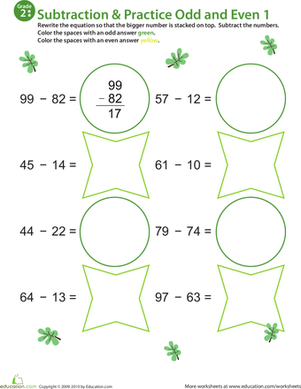### Maths practise: Subtraction Odd/Even 1

This year three maths practise worksheet is a double whammy. She'll solve each subtraction problem, and then determine whether the answers are odd or even.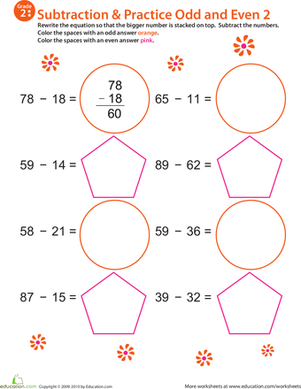### Maths Mania: practise Subtraction & Odd/Even 2

Give your second grader extra practise with both subtraction and determining which numbers are odd and even with this colorful worksheet!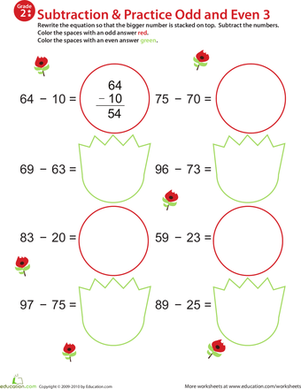### Maths Mania: practise Subtraction & Odd/Even 3

This bright worksheet is sure to keep your second grader entertained as he practices important concepts such as subtraction and odd/even numbers.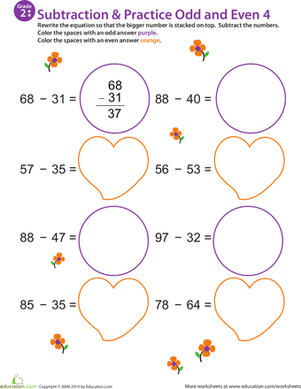### Maths Mania: practise Subtraction & Odd/Even 4

This colorful worksheet will give your second grader practise with double-digit subtraction while also getting her to identify odd and even numbers.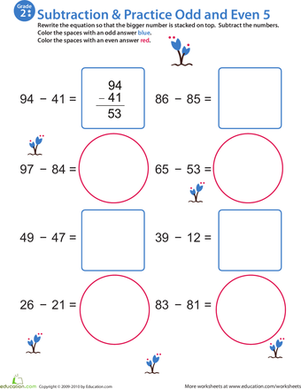### Maths Mania: practise Subtraction & Odd/Even 5

This colorful printable encourages your second grader to practise subtraction as well as odd and even numbers!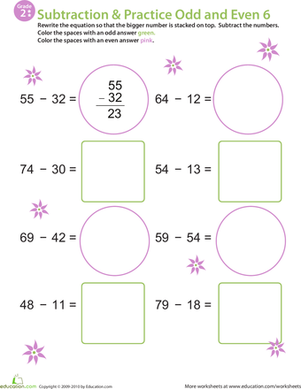### Maths Mania: practise Subtraction & Odd/Even 6

Here's a fun and colorful way to teach your second grader important concepts: subtraction and odd/even numbers.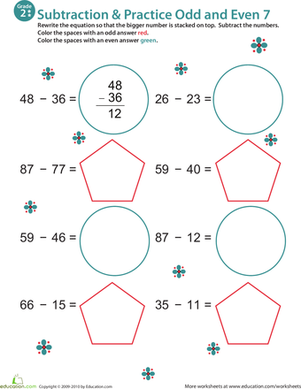### Maths Mania: practise Subtraction & Odd/Even 7

Ask your second grader to practise double-digit subtraction and encourage practise with odd and even number identification with this colorful worksheet!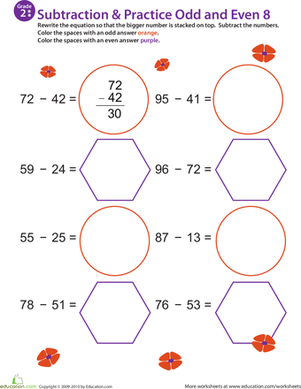### Maths Mania: practise Subtraction & Odd/Even 8

Challenge your second grader to master subtraction as well as odd and even numbers with this fun printable!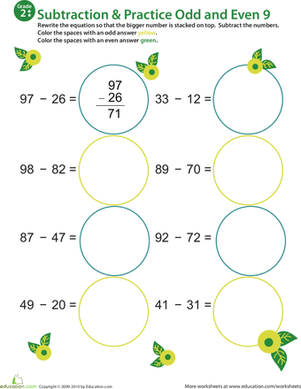### Maths Mania: practise Subtraction & Odd/Even 9

On the surface, this worksheet is all about double-digit subtraction, but it also challenges your second grader to practise identifying odd and even numbers!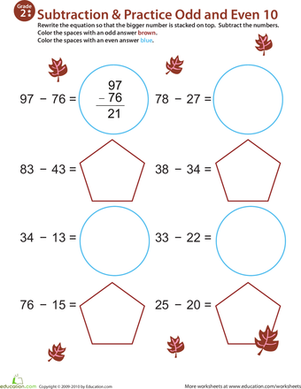### Maths Mania: practise Subtraction & Odd/Even 10

Boost your second grader's maths skills without boring him! Challenge him to solve seven subtraction problems, and then identify which answers are odd and even.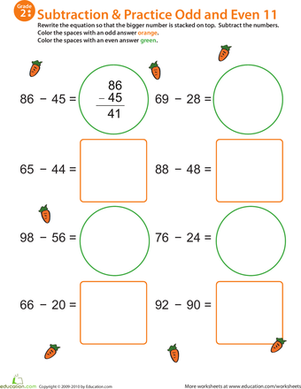### Maths Mania: practise Subtraction & Odd/Even 11

Challenge your second grader to finish this double-whammy worksheet; it includes double-digit subtraction as well as odd and even number identification.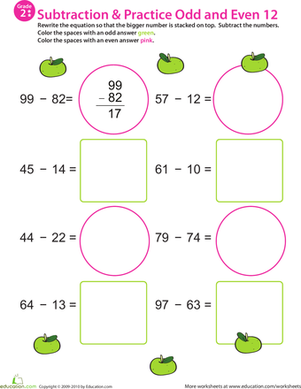### Maths Mania: practise Subtraction & Odd/Even 12

Don't let your second grader's maths practise go to waste! This double-digit subtraction exercise also challenges her to identify odd and even numbers.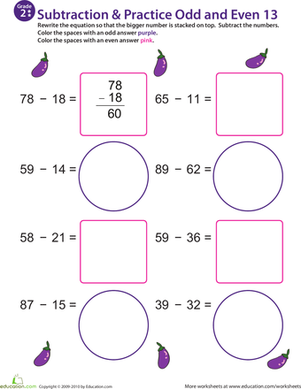### Maths Mania: practise Subtraction & Odd/Even 13

Boost your second grader's subtraction skills and ability to identify odd and even numbers with this colorful 2-in-1 worksheet!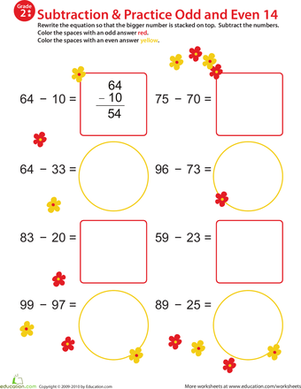### Maths Mania: practise Subtraction & Odd/Even 14

Incorporate both double-digit subtraction practise and an exercise identifying odd and even numbers into your second grader's maths practise.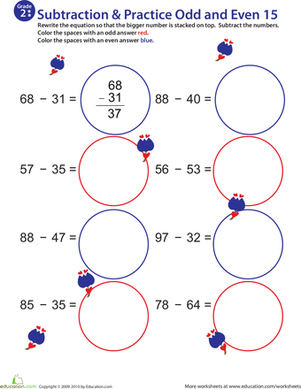### Maths Mania: practise Subtraction & Odd/Even 15

This year three printable provides a great way to maximize maths time; it incorporates double-digit subtraction as well as odd and even number identification.

Create new collection

0

### New Collection>

0Items

What could we do to improve Education.com?